## Monday, 17 September 2012

### CBSE Class 7 - Science - CH13 - Motion and Time

Motion and Time
MCQs, NCERT Chapter Solution, Q & A

Q1(MCQ/NCERT): The basic unit of speed is:
(a) km/min
(b) m/min
(c) km/h
(d) m/s

Answer: (d) m/s

Q2(MCQ): A faster moving object covers:

(a) Less distance in more time
(b) More distance in more time
(c) Less distance in shorter time
(d) More distance in shorter time

Answer: (d) More distance in shorter time

Q3(MCQ): If the an object moving along a straight line, keeps changing its speed then,
(a) The motion is said to be a uniform motion
(b) The motion is said to be a non uniform motion
(c) The object is said to be stationary
(d) The motion is said to be a fast motion

Answer: (b) The motion is said to be a non uniform motion

Q4(MCQ/NCERT): Which of the following relations is correct?

(a) Speed = Distance × Time
(b) Speed = Distance ÷ Time
(c) Speed = Time ÷ Distance
(d) Speed = 1/(Distance × Time)

Answer: (b) Speed = Distance ÷ Time

Q5(MCQ/NCERT): A car moves with a speed of 40 km/h for 15 minutes and then with a speed of 60 km/h for the next 15 minutes. The total distance covered by the car is:
(a) 100 km
(b) 25 km
(c) 15 km
(d) 10 km

Answer: (b) 25 km
Distance = Speed × Time
15 minutes = 15/60 = 0.25 hours
Distance Covered in first 15 min or 0.25 hours (D1) = 40 × 0.25 =10.00 km
Distance Covered in next 15 min or 0.25 hours (D2) = 60 × 0.25 =15.00 km
Total Distance Covered = D1 + D2 = 10 + 15 = 25 km

Q6(MCQ): 72 km/hr is same as

(a) 72 m/s
(b) 7.2 m/s
(c) 200 m/s
(d) 20 m/s

Answer: (d) 20 m/s
72km/hr = 72  × 1000m / 3600 seconds = 20m/s

Q7(MCQ/NCERT): Which of the following distance-time graphs shows a truck moving with speed which is not constant?

Answer: (c).

Q8(NCERT): Classify the following as motion along a straight line, circular or oscillatory motion:
(i) Motion of your hands while running.
(ii) Motion of a horse pulling a cart on a straight road.
(iii) Motion of a child in a merry-go-round.
(iv) Motion of a child on a see-saw.
(v) Motion of the hammer of an electric bell.
(vi) Motion of a train on a straight bridge.

Answer:
(i)   Oscillatory
(ii)  Straight Line
(iii) Circular
(iv) Oscillatory
(v)  Oscillatory
(vi) Straight Line

Q9(MCQ): The time interval between two consecutive sunrises is known as a
(a) Month
(b) Year
(c) Day
(d) Interval

Answer: (c) Day

Q10NCERT): Which of the following are not correct?
(i) The basic unit of time is second.
(ii) Every object moves with a constant speed.
(iii) Distances between two cities are measured in kilometres.
(iv) The time period of a given pendulum is not constant.
(v) The speed of a train is expressed in m/h.
(vi) A nanosecond is one billionth of a second.
(vii) The time taken by the pendulum to complete one oscillation is called its time period.
(viii) An hourglass is used to measure the movement of the Sun to compute time.

Answer:
(i) True (✓)
(ii) False (✗) Different objects have different speeds.
(iii) True (✓)
(iv) False (✗) The time period of a given pendulum is fixed.
(v) False (✗) The speed of train is usually expressed in km/hr or miles/hr.
(vi) True (✓)
(vii) True (✓)
(viii) False (✗)

Q11NCERT): A simple pendulum takes 32 s to complete 20 oscillations. What is the time period of the pendulum?

Answer: Time Period = Total Time Taken ÷ No. of Oscillations
No. of Oscillations = 20
Total Duration =  32s
Time Period = 32 ÷ 20 = 1.6s

Q12(NCERT): The distance between two stations is 240 km. A train takes 4 hours to cover this distance. Calculate the speed of the train.

Answer: Distance between two stations = 240 km
Time taken by train to cover the distance = 4 hours
Speed = Distance ÷ Time
Speed of Train = 240 / 4 = 60 km/hour.

Q13(NCERT): The odometer of a car reads 57321.0 km when the clock shows the time 08:30 AM. What is the distance moved by the car, if at 08:50 AM, the odometer reading has changed to 57336.0 km? Calculate the speed of the car in km/min during this time. Express the speed in km/h also.

Answer: Odometer reading at 8.30 AM ( O1) = 57321.0 km
Odometer reading at 8.50 AM (O2) = 57336.0 km
Distance covered by car =  O2 - O2 = 57336.0 - 57321.0 = 15 Km

Time interval between 8.30 AM to 8.50 AM = 20 min.

Speed of car = Distance ÷ Time = 15km ÷ 20 min = 0.75 km/min
1 Hr = 60 min.
Speed of Car in km/hr  = 0.75 × 60 = 45 km/hr.

Q14(NCERT): Salma takes 15 minutes from her house to reach her school on a bicycle. If the bicycle has a speed of 2 m/s, calculate the distance between her house and the school.

Answer: Distance = Speed × Time
Speed of the bicycle  = 2 m/s
Time taken to reach school = 15 min = 15 × 60seconds = 900s
Distance = 2 × 900 = 1800 m = 1800/1000Km = 1.8 Km

Q15(NCERT): Show the shape of the distance-time graph for the motion in the following cases:
(i) A car moving with a constant speed.
(ii) A car parked on a side road.

Answer:
(i) A car moving with a constant speed.
When a car moves with a constant speed, it will cover equal distance in equal intervals of time. In a distance-time graph it is a straight line inclined to time-axis.

(ii) A car parked on a side road.
A car parked means, the car is not moving at all. It is at rest. The distance-time graph will be a horizontal line parallel to time-axis.

Q16(NCERT): Suppose the two photographs, shown in Fig. A and Fig. B below, had been taken at an interval of 10 seconds. If a distance of 100 metres is shown by 1 cm in these photographs, calculate the speed of the blue car.

Answer: 1 cm = 100 m
Distance covered by blue car = 2.0 cm = 2.0 × 100 = 200 m
Time taken to cover 200m = 10s
Speed of Car =  Distance ÷ Time = 200 / 10 = 20m/s
Speed of Car (in km/hr) = 20 × 3600s/1000m = 72 km/hr

Q17(NCERT): Figure below shows the distance-time graph for the motion of two vehicles A and B. Which one of them is moving faster?

Answer: The steeper the line, greater the speed. Vehicle A moves faster than B.

Q18: Rohan covers a distance of 10 km by running with uniform speed of 4.5 km/hr. Sohan runs 1.2 km in 15 minutes. Who runs faster?

Answer: Let us compare the speed of these two persons.
Rohans's speed = 4.5 km/hr
15 mins = 15/60 = 0.25 hrs
Sohan's Speed = Distance/Time = 1.2km/0.25 = 1.2 × 4 = 4.8 km/hr
Therefore, Sohan's speed is higer than Rohan.

Q19: Give Reasons:
1. Accurate measurements of time became possible much after accurate measurements of length and mass.
2. The time measured by quartz clocks is much more accurate than that by the clocks available earlier.
3. All clocks make use of some periodic motion.
4. Speed is called scalar quantity.
5. Displacement is called vector quantity.
6. Average speed is same as actual speed in case of a body having uniform motion.
Answer:
(a)  Accurate measurements of time became possible much after accurate measurements of length and mass.
Length and mass were the earliest measurements made mankind. Time measurement was done in terms of length as distance and mass. For example position of sun, moon and stars give idea of days, months and years. Later mechanical clocks based on weights and spring can measure time accurately up to minutes. Today atomic clocks measures time accurate upto nano-seconds by measuring waves emitted by Cesium clock in terms of speed of light and frequency. Over the time improvements in accuracy in measuring length and mass help in measuring time with more accuracy and precision.

(b) The time measured by quartz clocks is much more accurate than that by the clocks available earlier.
Quartz crystals emit vibrations are fixed frequency. These vibrations (pulses) are counted and are used to measure time. It can measure time up to milliseconds and has high accuracy i.e. a normal quartz wristwatch may lose or gain less than a fraction of a second in a day. While the mechanical clocks used earlier were based on weights and spring which rotate the gears. The lowest time interval these mechanical clocks is up to minute. Hence accuracy of quartz clocks is more accurate than the clocks of earlier times.

(c) All clocks make use of some periodic motion.
The working principle of all clocks is same i.e. periodic motion. A sundial measures the periodic position of shadow on a dial.A pendulum clock measures number of oscillations of the bob. A spring clock measures back and forth motion of the spring. A quartz clock measures the number of vibrations or oscillations of the quartz crystal.

(d) Speed is called scalar quantity.
Speed tells how much distance is travelled by a body per unit time. It is simply a numerical value and does not tell the direction of the moving object. ∴ speed is a scalar quantity.

(e) Displacement is called vector quantity.
Displacement has both magnitude and direction. e.g. John's car is now 450m away from here in north direction. ∴ displacement is a vector quantity.

(f) Average speed is same as actual speed in case of a body having uniform motion.
Uniform motion means the object covers equal distances in equal intervals of time.
Average speed means total distance covered in total time.

Consider an object in uniform motions goes from A to B in 5 seconds and then B to C in 5 seconds. Let AB = BC = 20m.

Actual speed of the object (A to B) or (B to C) = Distance ÷ time = 20m ÷ 5s = 4m/s

Average Speed = Total Distance ÷ Total Time = (20m + 20m) ÷ (5s + 5s) = 40m ÷ 10s = 4m/s

Thus Average speed is same as actual speed in case of a body having uniform motion.#### 24 comments:

1.how to find time period

1.e.g. measure time 't' for 25 oscillations using a stop watch. Average Time Period T = t/25

2.In question 6
m = miles
or m = metres

1.2.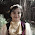3.in q:18-why to multiply 1.2 x 4

4.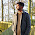in question 16
1cm=100m is wrong
only
1m=100cm is correct

1.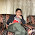Are you out of your mind. They have just denoted 1 cm as 100m
It's not wrong.

2.no baccha navaneetha is correct they should denote it like that

5.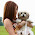Hi GunaSekaran Muthukrishnan! I think this article contain some errors. you can see these errors in the comments. any way i agree with you that in Question No. 18 we multiply 4.5 instead of 4. I mean 4.5*1.2. Are you agree with me. Last night i have found a math website which i like to share here. This website name is Math Fractions. This helpful website for math.

6.7.Nice, But improvement needed

8.yes, in Q16.It was written wrong that 1cm=100m,
it is 1m=100cm. because we are calculating speed of the blue car.

9.get lost . it was of no use for me
:P

10.an aircraft flying at a constant speed is an example of
1. periodic motion
2.random motion
3. uniform motion
4. non uniform motion

answer the true one

11.no use for me this is a bekar site

12.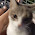was really hepful

13.was really hepful

14.no motion known as "straight line" motion its rectillinear motion

15.In this chapter answer this question:-
Explain why we need to measure time?

16.It's very helpful

17.a train covers 115000 meter in 4200 seconds.calculate its speed in km/hr

18.i love my brother madhav seth he did a lot o hard work and is now in the fist year of his collage in usa named UC SANDIAGO i also want to go and study in usa so i want to be more intelgent and choose this thing it is easy but some of the Q are tricky so i want thatCBSE people should tell the school teachers of delhi to give a very easy pap
er in science test

19.This comment has been removed by the author.

We love to hear your thoughts about this post!